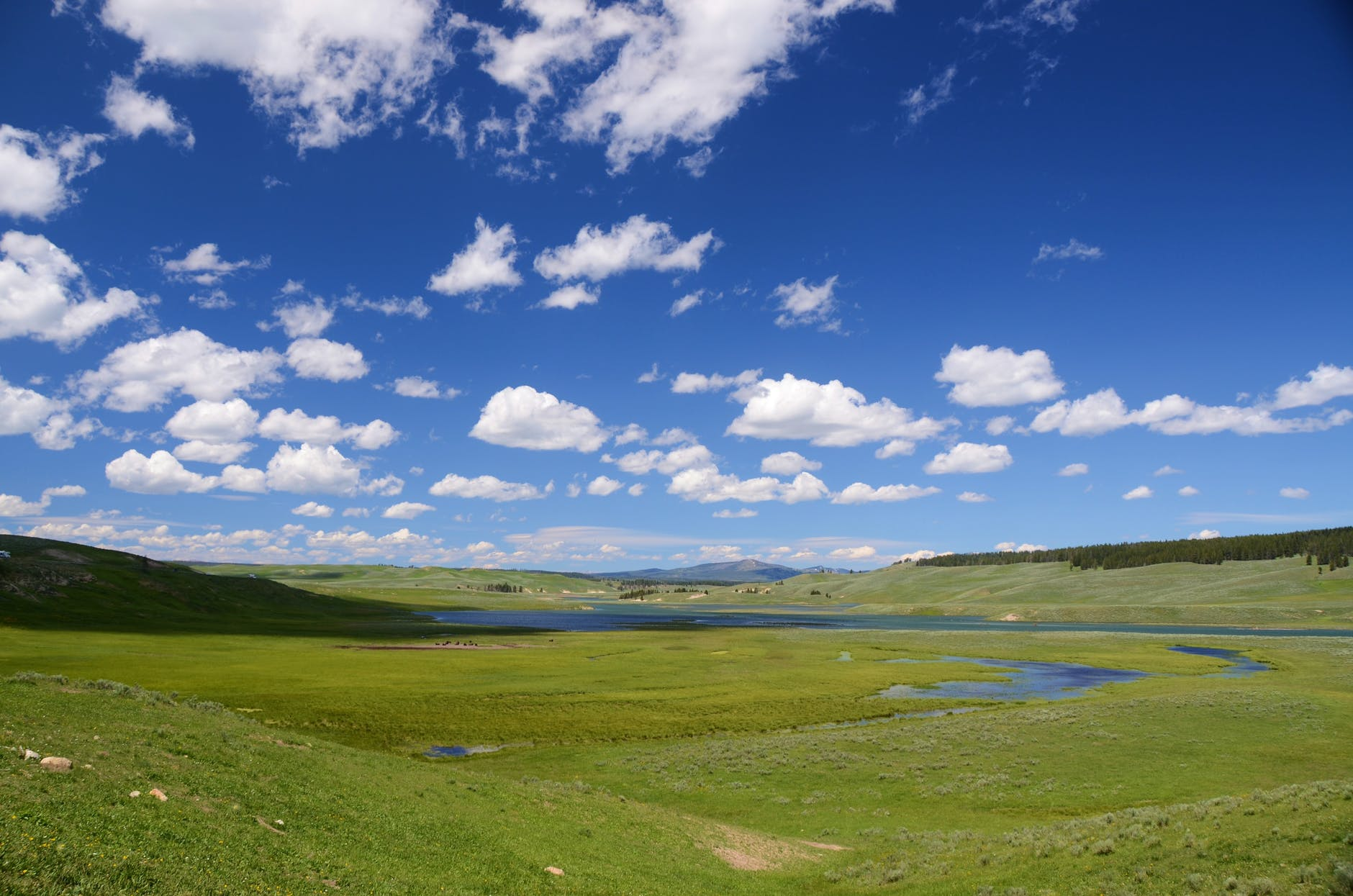# Week 32

Example 1. First 100 natural numbers are written on a black board. Two persons A and B are playing a game of putting + and – sign one by one between any two consecutive integers out of these 100 natural numbers. Both A and B are free to put any sign (+ or –) anywhere provided there is no sign placed already between the two natural numbers. At the end, when the signs are put between all such two consecutive natural numbers, result is calculated. If the result is even, then A wins and if the result is odd, then B wins. Who will win?

Example 2. Given that cos x + cos y + cos z + cos w = 4. Find the value of sin2 x + sin3 y + sin4 z + sin5 w.

Of course, you can find the solution just below, but it is highly recommended that, you first try to solve it on your own.

Just remember the words of Paul Halmos, who says “the only way to learn mathematics is to do mathematics”.

Solution 1. Among 100 natural numbers, there are 50 odd and 50 even numbers.

So, 100 consecutive natural numbers = set of 50 odd and 50 even numbers.

Whatever sign we put between two odd numbers, resultant of 50 odd numbers = Even, and similarly, whatever sign we put between two even numbers, resultant of 50 even numbers = Even.

Hence the net result is either Even + Even or Even – Even.

In any case, net result = Even. Hence A will win.

We can also see here that + sign or – sign does not matter here. Irrespective of the signs put by A or B, A is always going to win.

Solution 2. Given that cos x + cos y + cos z + cos w = 4.

Here x, y, z and w are angles. We need to apply the maximization theory.

Since −1 ≤ cos t ≤ 1, maximum value of cos t is 1.

Therefore, cos x + cos y + cos z + cos w = 4 = 1 + 1 + 1 + 1 is possible only when

cos x = 1, cos y = 1, cos z = 1 and cos w = 1.

In other words, when x = y = z = w = 0.

In that case, sin x = sin y = sin z = sin w = 0.

Hence sin2 x + sin3 y + sin4 z + sin5 w

= sin2 (0) + sin3 (0) + sin4 (0) + sin5 (0)

= 0 + 0 + 0 + 0

= 0.

1.zoritoler imol says:
2.Copy paintings of famous artists says: